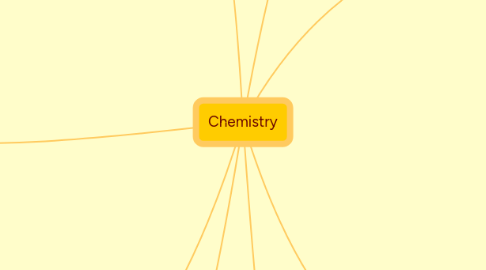# Chemistry

Chemistry Mind Map

Get Started. It's FreeChemistry## 1. Chemical Quantities

### 1.2. Mole mass and Mole volume relationships

1.2.1. mol = representative particles/avagadros number

1.2.2. mol = mass/ molar mass

1.2.3. mol = volume/ molar volume

## 2. Acids and Bases

### 2.7. pH + pOH = 14

2.7.1. [H+][OH-] = 10E-14 at 25C